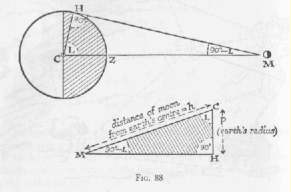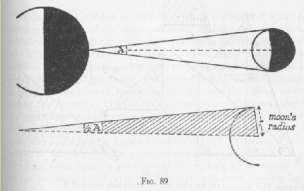Homework 2 - Physics 150 Fall 2003
Due September 17, 2003

1. Iamgine yourself living 7000 years ago in an advanced civilization such as Egypt. Write arguments for or against each of the three propositions below.
a. The Earth is at the center of the universe.
b. The Sun is at the center.
c. There is no "center".
2. Questions/Problems in the text, March
Chap. 2, Question C

Chap. 2, Problem 2

Chap. 2, Problem 4

Chap. 4, Question B

Remember that there are hints, worked exercise that covers several examples, and there are answers to odd-numbered problems that are very similar to problems assigned.
3. In thes exercises you are asked find the distance of the moon from the earth and the size of the moon using ideas that were developed in the classsical period of Greece.Assume you know the radius of the earth (use the modern known value), and you make the measurement shown in the picture, finding the angle L to be L = 89.1 degrees. From this information what is the calculated distance of the moon to the earth?

4. Now that we know the distance from the moon to the earth, we can calculate the diameter of the moon, according to the picture below.Assume you have measured A = 0.5 degree. Calculate the diameter of the moon. How does it compare to the real value?

5. Comment on the accuracy of such measurements, the difficulty that the ancients would have had in making these measurements, and the need for accurate values of pi and of the sine of the angles.
Pictures from: "Mathematics for the Million"